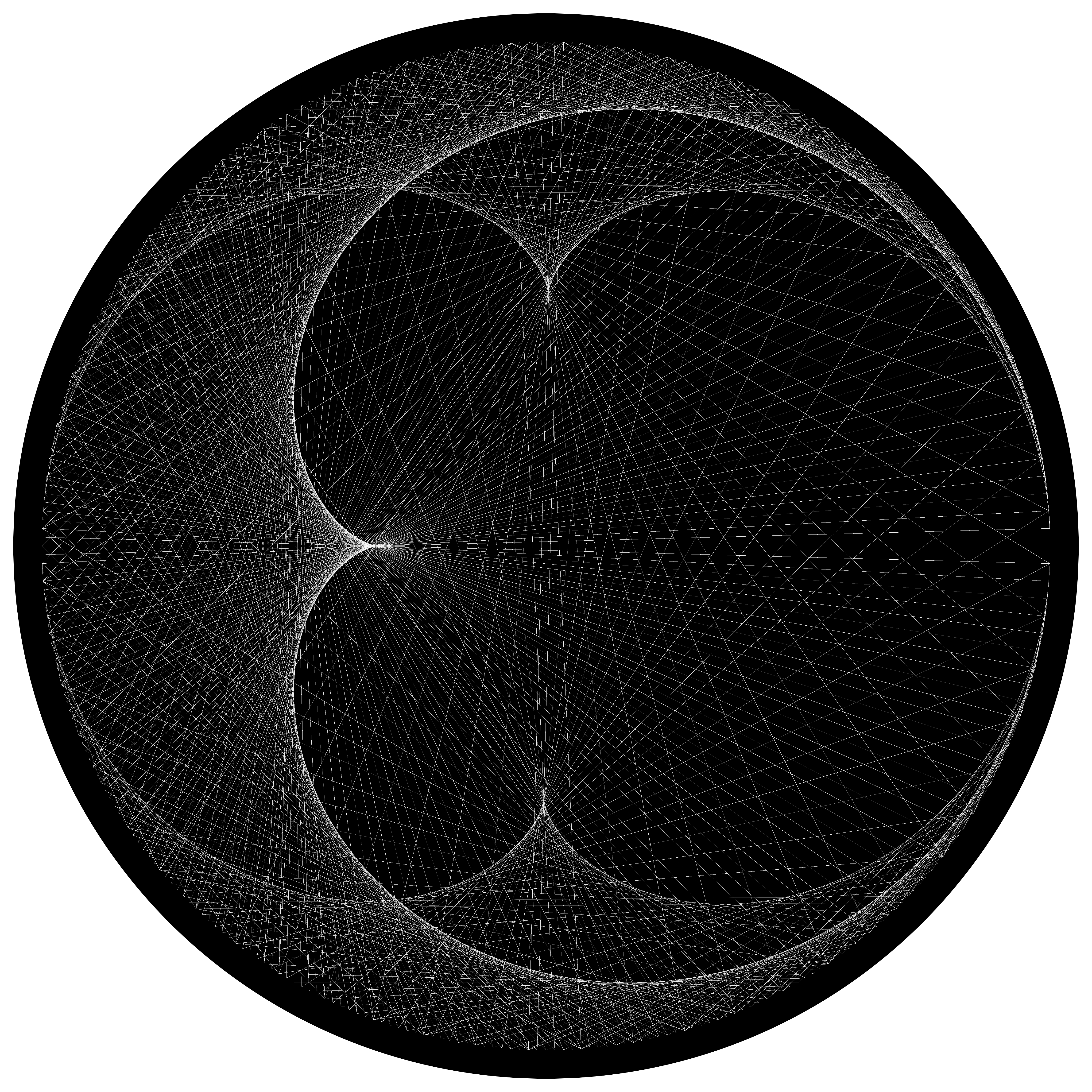# Plotting the first 2,000 Collatz sequences in polar coordinates produces a cardioid and a nephroid

Written on January 30, 2021

The Collatz conjecture is a well-known math problem that concerns the following sequence for a given starting integer N:

• If the number is even, divide it by 2.
• If the number is odd, multiply it by 3 and add 1.

And then repeat this operation, forming a sequence.

For example, for the starting integer of 5:

• 5 is odd, so we multiply it by 3, giving us 16.
• 16 is even, so we divide it by 2, giving us 8.
• 8 is even, so we divide it by 2, giving us 4.
• 4 is even, so we divide it by 2, giving us 2.
• 2 is even, so we divide it by 2, giving us 1.

If we were to continue, we would loop back from 1 to 4 to 2 to 1 indefinitely, so we terminate the sequence upon reaching 1. So for the Collatz sequence for 5, we can write: 5 -> 16 -> 8 -> 4 -> 2 -> 1.

The Collatz conjecture states that for every starting integer, the sequence will eventually reach 1. The conjecture is yet unproven but every starting number up to 5.764 x 10^18 has been checked and confirmed that they do, in fact, reach 1.

Below, I generate the Collatz sequences for the first 2,000 starting integers and plot them in polar coordinates, using a fixed radius and with θ equal to the integer (converted to radians, so effectively with modulo 360).Each sequence is plotted independently on top of each other, with each consecutive point in each of the 2,000 sequences connected by a (semi-transparent) line. The total number of elements in the 2,000 sequences is 136,100, so the total number of lines plotted in the image below is 136,100 - 2,000 = 134,100. (The code that generates the image is available here.)

We can see two clear shapes – a cardioid overlaid on a nephroid.

Now, why do these shapes appear? My experience in number theory only takes me this far, so I’ll have to hang up my spurs here, but will be sending the question out to a couple more knowledgable parties. As of now, there’s no substantive references online to nephroids and the Collatz conjecture together. I’ll update this post with any progress!

UPDATE (February 10, 2021):

See Part 2.